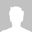Extended 8+1 = 9-Bit SPI decoding

I have to debug a weird SPI protocol where 8 data-bits are followed by an unused 9th bit (similar to parity bit). It would be great to have an additional SPI-protocol in the format 8+1, where you can choose not to show the 9th bit.

Another - more general - way would be, to introduce separate calculation channels with some basic math, where you can do calculations on the output of the analysers. In my case, I could insert a calculation like this: " myCalculationChannel = MOSI >> 1 " (i.e. shift right by one bit).

•Guest
• Jun 26 2018